5G时代下一个聚合的编程学习网

# 栈的压入弹出序列

question：输入两个整数序列，第一个序列表示栈的压入顺序，请判断第二个序列是否可能为该栈的弹出顺序。假设压入栈的所有数字均不相等。例如序列1,2,3,4,5是某栈的压入顺序，序列4,5,3,2,1是该压栈序列对应的一个弹出序列，但4,3,5,1,2就不可能是该压栈序列的弹出序列。（注意：这两个序列的长度是相等的）

``````import java.util.ArrayList;

public class Solution {
public boolean IsPopOrder(int [] pushA,int [] popA) {

}
}``````

annalysis: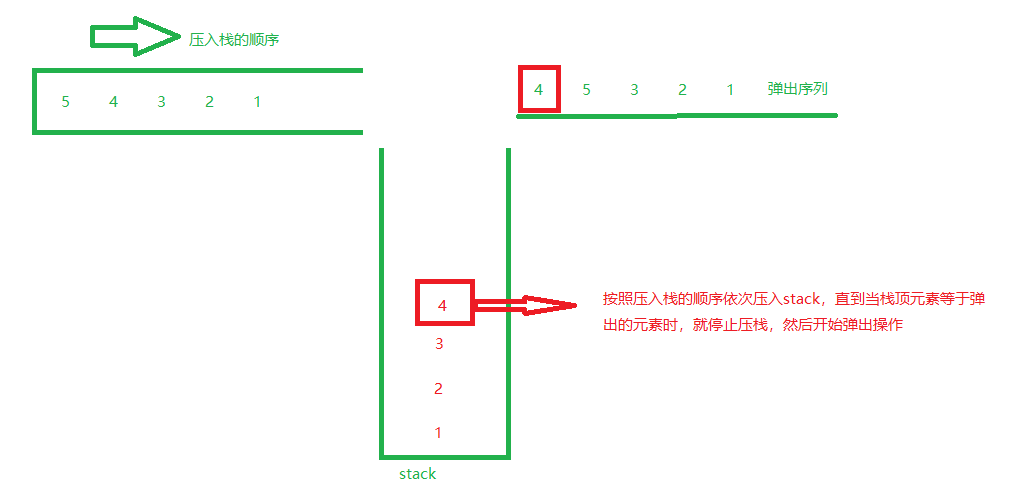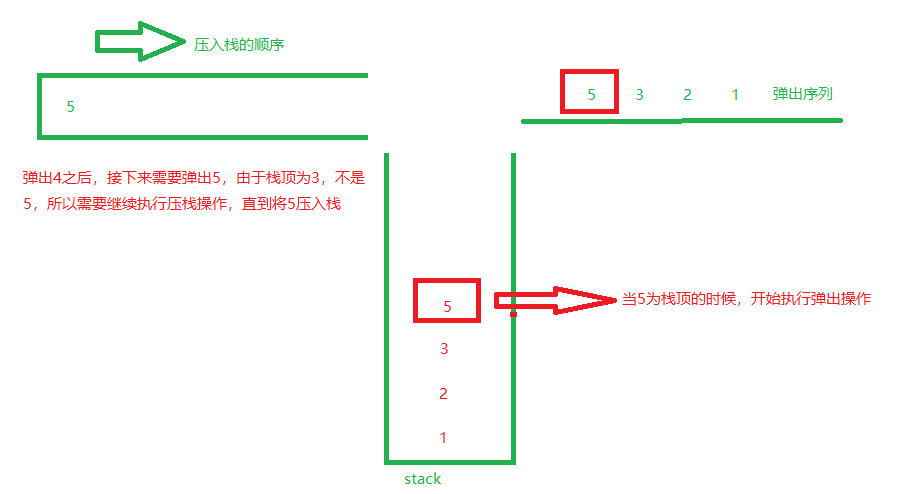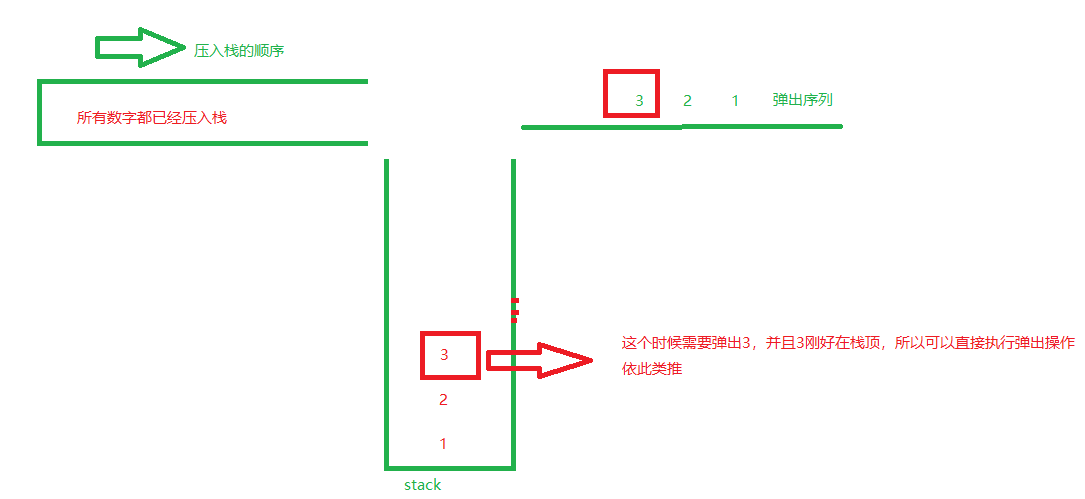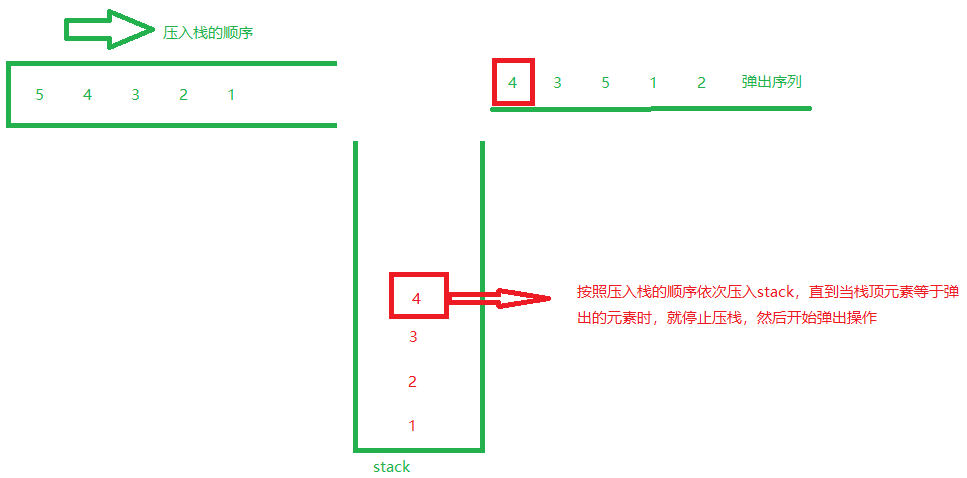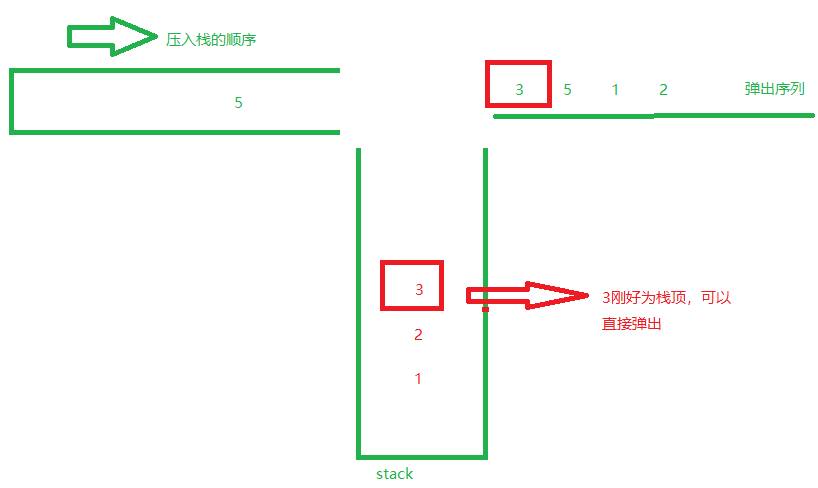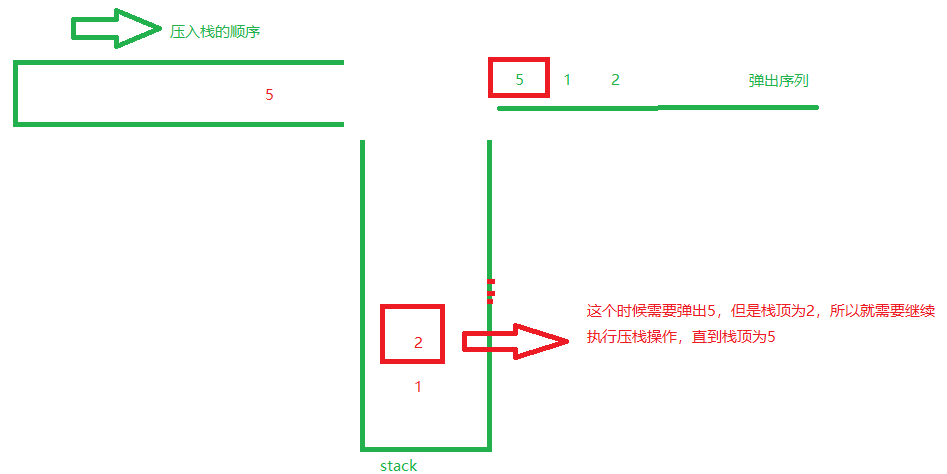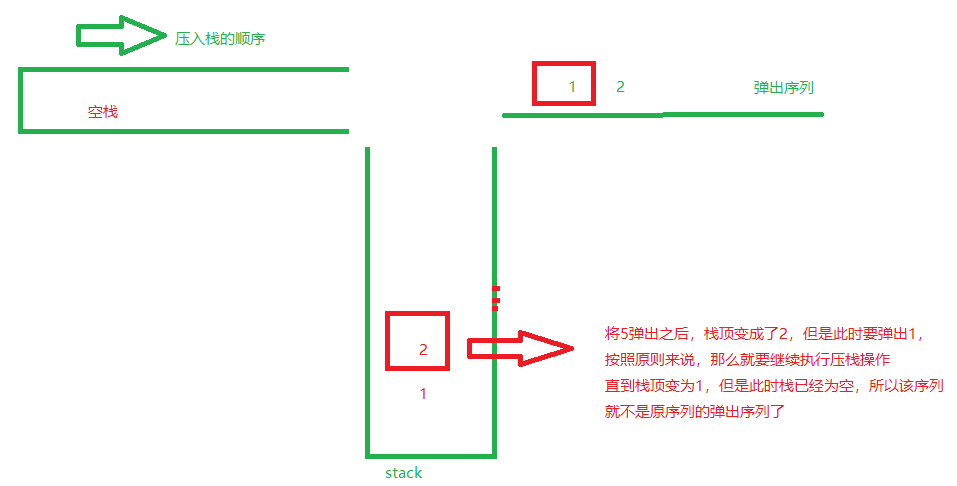resolution：

``````   public boolean IsPopOrder(int [] pushA,int [] popA) {
//创建两个栈分别存储压栈序列和弹出序列
Stack<Integer> stack = new Stack<Integer>();
Stack<Integer> popStack = new Stack<Integer>();
boolean flg = false;

//处理特殊情况
if(pushA.length == 0 || popA.length == 0 ) return flg;

//将popA数组倒序存入弹出栈（因为栈为后进先出，先进后出）
for(int i = popA.length - 1; i>=0;i--){
popStack.push(popA[i]);
}

//首先先将第一个数压入栈stack
stack.push(pushA);
//这里i的初值为1
int i = 1;

//设置终止条件为stack不为空
while(!stack.isEmpty()){
//处理当stack为空的时候的栈顶值，否则会抛出异常
//stack==null时不能调用stack.peek()方法
int stackPeek = 0;
if(stack.isEmpty()){
stackPeek = 0;
}else {
stackPeek = stack.peek();
}
int popStackPeek = popStack.peek();

if(stackPeek != popStackPeek){
//处理特殊情况
if(i == pushA.length) break;
stack.push(pushA[i]);
i++;

}

if(stack.peek() == popStack.peek()){
stack.pop();
popStack.pop();
//当弹出栈弹完了说明能够对应，返回true
if(popStack.isEmpty()) flg = true;
}
}

return flg;

}``````

``````  /**
* Looks at the object at the top of this stack without removing it
* from the stack.
*
* @return  the object at the top of this stack (the last item
*          of the <tt>Vector</tt> object).
* @throws  EmptyStackException  if this stack is empty.
*/
public synchronized E peek() {
int     len = size();

if (len == 0)
throw new EmptyStackException();//可以看出当len==0时，会抛出异常
return elementAt(len - 1);
}``````